# pylops.utils.seismicevents.hyperbolic2d¶

pylops.utils.seismicevents.hyperbolic2d(x, t, t0, vrms, amp, wav)[source]

Hyperbolic 2D events

Create 2d hyperbolic events given intercept time, root-mean-square velocity, and amplitude of each event

Parameters: x : numpy.ndarray space axis t : numpy.ndarray time axis t0 : intercept time at $$x=0$$ of each of hyperbolic event vrms : root-mean-square velocity of each hyperbolic event amp : amplitude of each hyperbolic event wav : numpy.ndarray wavelet to be applied to data d : numpy.ndarray data without wavelet of size $$[n_x \times n_t]$$ dwav : numpy.ndarray data with wavelet of size $$[n_x \times n_t]$$

Notes

Each event is created using the following relation:

$t_i(x) = \sqrt{t_{0,i}^2 + \frac{x^2}{v_{\text{rms},i}^2}}$

## Examples using pylops.utils.seismicevents.hyperbolic2d¶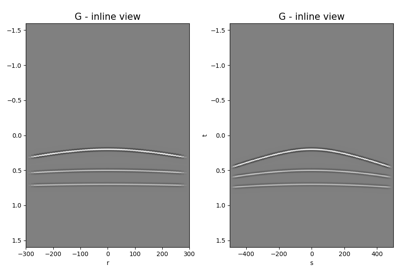Multi-Dimensional Convolution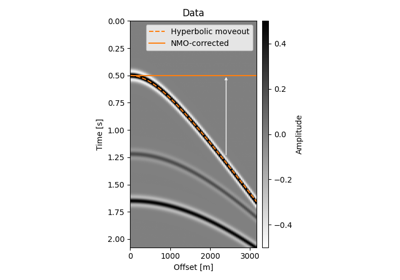Normal Moveout (NMO) Correction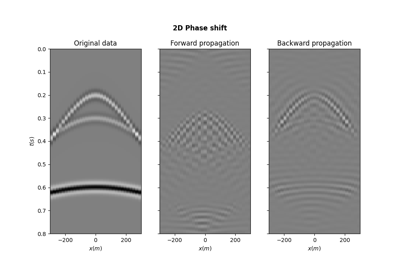PhaseShift operator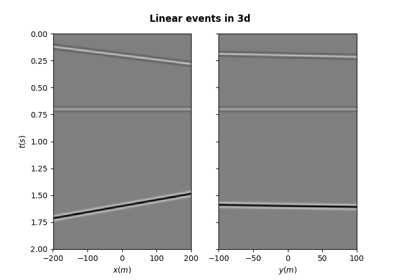Synthetic seismic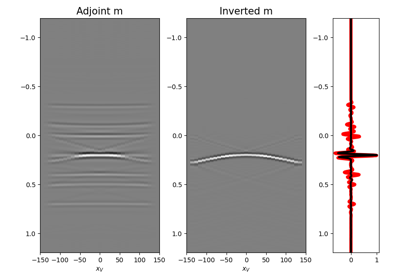09. Multi-Dimensional Deconvolution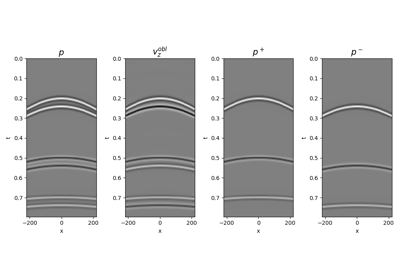14. Seismic wavefield decomposition